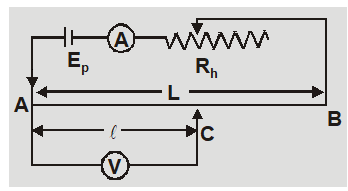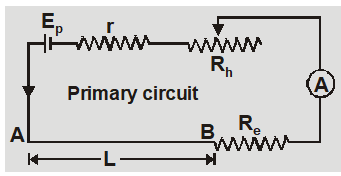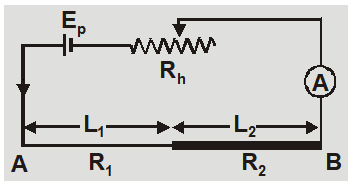Download the eSaral app and start learning from Kota's top IITians and doctors.

# Potentiometer Working Principle, Uses, Advantages & Disadvantages || Class 12, JEE & NEET

A potentiometer is a device used to measure the potential difference in a circuit. As we know that potential difference is the amount of work done in bringing a charge from one point to another. When there is potential difference in a circuit, the current flows through the circuit. The Unit of Potential Difference is measured in Volts. The potential difference of a circuit can be measure by a voltmeter. Now we will see Potentiometer Working Principle with the uses of Potentiometer.

## Potentiometer Working Principle

The potential drop across any section of wire of uniform cross-section and composition is proportional to length of that section if a constant current flows through it. If $\mathbf{I}$ is the current in potentiometer wire AB of uniform cross-sectional area A, length L and specific resistance $\rho$ then unknown potential difference across AC is $V =\frac{ I \rho \ell}{ A }$ and known potential difference across AB is $E_{p}=\frac{I \rho L}{A}$At balance point unknown potential difference = known potential difference or $\frac{V}{\ell}=\frac{E_{p}}{L}$ or $V=\left(\frac{E_{p}}{L}\right) \ell$ or $V=x l$ $$\text { so } \quad V \propto \ell$$ where x = $E_{p} / L$= potential gradient i.e. fall of potential per unit length of potentiometer. Important Points
1. Potentiometer was devised by Poggendorf.
2. The positive terminals of driving cell and unknown cell must be connected to the same end of potentiometer wire to obtain a balance point.
3. A balance point is obtained on potentiometer wire if the fall of potential along potentiometer wire due to driving cell $E_{p}$ is greater than emf of cell to be balanced.
4. The diameter of potentiometer wire must be uniform, specific resistance $P$ must be large and temperature coefficient  of material of wire must be small.
5. It is based of deflection method i.e. while measuring emf it does not draw any current from source of driving emf.
6. While measuring unknown potential difference the resistance of potentiometer wire becomes infinite.

### Browse More Topics Related to Potentiometer:

The fall of potential per unit length of potentiometer wire is called potential gradient. r = internal resistance of driving cell; $R_{h}$ = resistance of rheostat, $R_{e}$ = external series resistance, R is resistance of potentiometer wire, L is length of potentiometer wire. The current through primary circuit $I=\frac{E_{p}}{r+R_{h}+R_{e}+R}$#### The potential gradient $x=\frac{I R}{L}=\frac{E_{p}}{r+R_{h}+R_{e}+R}\left(\frac{R}{L}\right)$

1. If $R_{h}$ = 0 and $R_{e}$ = 0 x = $X_{\max }$ = $\frac{E_{p} R}{(r+R) L}0024 2. x =$\mathrm{X}_{\mathrm{min}}$=$\frac{E_{p}}{R+R_{h}+R_{e}+R}\left(\frac{R}{L}\right)$3.$x =\frac{ V }{ L }=\frac{\text { current } \times \text { resistance of potentiometer wire }}{\text { length of potentiometer wire }}= I \left(\frac{ R }{ L }\right)$4. where R/L is resistance per unit length of potentiometer wire. 5.$R=\frac{\rho L}{A}$or$\frac{ R }{ L }=\frac{\rho}{ A }$so$x =\frac{ I \rho}{ A }=\frac{ I \times \text { specific resistance of material }}{\text { area of cross - sec tion }}$6. Unit of potential gradient is volt/meter and dimensions are$M^{1} L^{1} T^{-3} A^{-1}$. 7. The potential gradient depends only on primary circuit and is independent of secondary circuit. 8. On increasing the temperature of potentiometer wire there is no change in potential gradient if a constant current is maintained. If current is altered due to change in resistance of wire then potential gradient will change. 9. Keeping the thickness of potentiometer wire constant if the length is changed from$L_{1}$to$L_{2}$then ratio of potential gradient will be$\frac{x_{1}}{x_{2}}=\frac{L_{2}}{L_{1}}$10. If two wires of length$\mathrm{L}_{1}$and$\mathrm{L}_{2},$resistances$\mathrm{R}_{1}$and$\mathrm{R}_{2}$are joined in series with a battery of emf$E_{p}$and a rheostat than the ratio of potential gradients can be calculated as$x_{1}=\left(\frac{E_{p}}{R_{1}+R_{2}}\right) \frac{R_{1}}{L_{1}} \quad$and$\quad x_{2}=\left(\frac{E_{p}}{R_{1}+R_{2}}\right) \frac{R_{2}}{L_{2}} \quad$or$\quad \frac{x_{1}}{x_{2}}=\frac{R_{1}}{R_{2}} \cdot \frac{L_{2}}{L_{1}}$The potential gradient depends on: 1. emf of battery in primary circuit$\left(E_{p}\right)$and its internal resistance (r). 2. Length of Potentiometer wire (L), its radius and its resistance (R). 3. Specific resistance of material of wire$(\rho)$. 4. Current flowing through the wire. 5. Additional resistance like resistance of rheostat$\left(R_{h}\right)$and series external resistance$\left(\mathrm{R}_{\mathrm{e}}\right)\$.